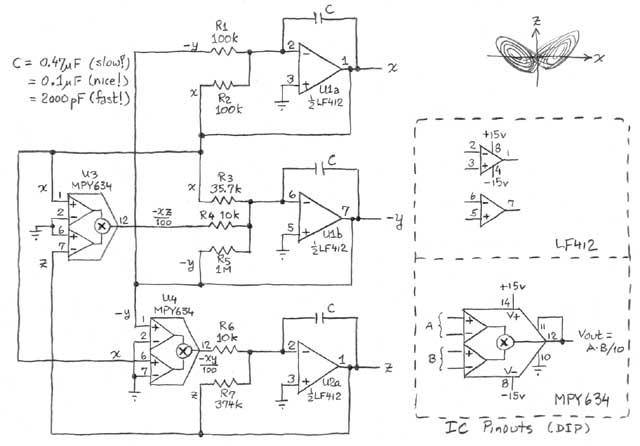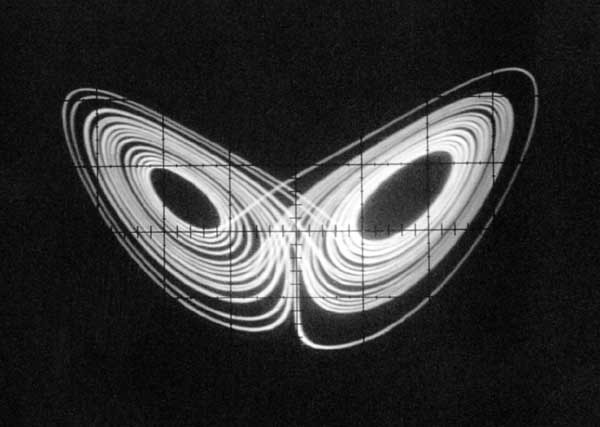## "Build a Lorenz Attractor"

```In 1963 Edward Lorenz published his famous set of coupled nonlinear
first-order ordinary differential equations; they are relatively simple,
but the resulting behavior is wonderfully complex.  The equations are:

dx/dt = s(y-x)
dy/dt = rx-y-xz
dz/dt = xy - bz

with suggested parameters s=10, r=28, and b=8/3.  The solution executes
a trajectory, plotted in three dimensions, that winds around and around,
neither predictable nor random, occupying a region known as its attractor.
With lots of computing power you can approximate the equations numerically,
and many handsome plots can be found on the web.  However, it's rather
easy to implement these equations in an analog electronic circuit, with
just 3 op-amps (each does both an integration and a sum) and two analog
multipliers (to form the products xy and xz).
```

### The Circuit

```Here's the circuit:
``````It's not hard to understand: the op-amps are wired as integrators, with
the various terms that make up each derivative summed at the inputs.  The
resistor values are scaled to 1 megohm, thus for example R3 weights the
variable x with a factor of 28 (1M/35.7k); this is combined with -y and
-xz, each with unit weight.  (note: the equations on the diagram are
normalized to 0.1V, hence the multiplier scale factor of 100.)
```

### The Output

```The circuit just sits there and produces three voltages x(t), y(t), and z(t);
if you hook x and z into a `scope, you get a pattern like this...
``````...the characteristic "owl's face" of the Lorenz attractor.  The curve
plays out in time, sometimes appearing to hesitate as it scales the
boundary and decides which basin to drop back into.  The value of C, the
three integrator capacitors, sets the time scale: at 0.47uF it does a
leisurely wander; at 0.1uF it winds around like someone on a mission; and
at 0.002uF it is fiercely busy solving its equations and delighting its
audience.
```

```For more info on how circuits work, and how to build them, you can't do
better than the highly readable, and even entertaining The Art of Electronics.

Back to Paul's page

```